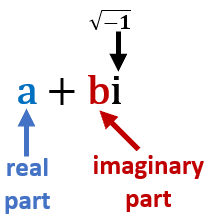# Math Worksheets Land

Math Worksheets For All Ages

# Math Worksheets Land

Math Worksheets For All Ages

Home > Topics >

# Complex Number System Worksheets

The concept of complex numbers was created as a tool to manipulate and understand unique types of equations that contain exponents. These particular equations caused mathematicians a great deal of difficulty because they produce numbers that when squared gave a negative result. Real numbers simply did not apply because negative values do not have a real square root. To tackle this mathematicians created something called an imaginary number which is often signified by the variable i. Imaginary numbers are values that when they are squared produce a negative result. Complex numbers are expressions that contain both real and imaginary numbers. Below you will see a wide offering of lessons and worksheets specific to this topic.

### What are Complex Number Systems?Complex numbers are one of the building blocks of advanced mathematics and are widely used in algebra. These numbers can be used in solving problems in real life, especially in electromagnetism and electronics.

Complex numbers are made up of two parts: an imaginary number and a real number. We are very familiar with real numbers they can be writing easily on a number line. They come in two forms as rational (can be written as a fraction) and irrational numbers (cannot be written as a fraction). Imaginary numbers are values that when they are squared result in a negative value.

The standard form of a complex number is written as 'a + bi', with 'a' being the real part and 'bi' being the imaginary part. You can see this displayed in the image to right. In these systems either part can be equal to zero. Therefore, any combination of imaginary or real number in this format can be considered as a complex number system. The value doesn't technically signify the intricacy of the system. It means that two types of numbers come together to form a complex.

In this system can consist of any tangible value such as integers, fractions, or countable numbers that can be graphed on the horizontal number line is considered as a real number. Imaginary numbers are more abstract concepts that are employed when the square root of a negative number is needed.

Complex numbers can also be plotted on the two-dimensional plane by plotting an imaginary number on the vertical axis and real numbers on the horizontal axis. the complex number can be denoted by points (a, b). They have many real world applications to model the movement of subatomic particles, modeling the frequency of sounds, and even to understand the relative strength and abilities of electrical circuits.

Unlock all the answers, worksheets, homework, tests and more!
Save Tons of Time! Make My Life Easier Now

## Thanks and Don't Forget To Tell Your Friends!

I would appreciate everyone letting me know if you find any errors. I'm getting a little older these days and my eyes are going. Please contact me, to let me know. I'll fix it ASAP.